Summing a range containing errors and values in Microsoft Excel

For summing a range containing errors and values, we can use the SUMIF  function or we can use  IF  function along with ISERROR function in Microsoft Excel.

SUMIF:This function is used to add the numbers in a range of cells on the basis of specific criteria.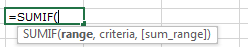SUM function is used to add the numbers in a range of cells.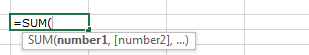IF: - Check if a condition is met and returns one value if True and another value if False.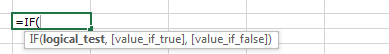ISERROR- This function is used for checking if a value is an error (#N/A, #VALUE!, #REF!, #DIV/0!, #NUM!, #NAME?, OR #NULL!) and returns True or False.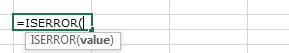Let’s take an example to understand how we can perform summing on a range containing errors and values in Microsoft Excel.

We have data in range A2:B11. Column A contains Agent list and column B contains sales amount; in column B, few cells contain #VALUE! Error. We want to return the total value to ignore the error.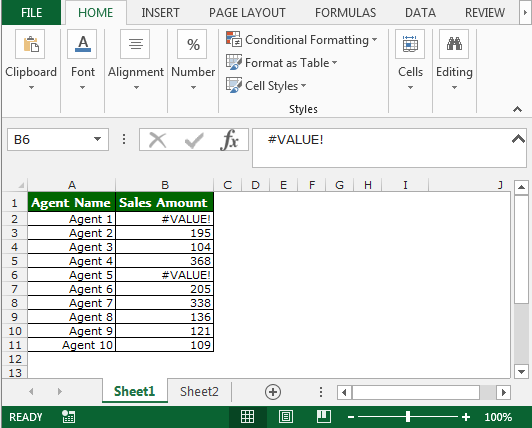• Select the cell C2.
• Write the formula =SUMIF(B2:B11,”<>#VALUE!”,B2:B11)
• Press enter on your keyboard.
• The function will return the total value; thereby, ignoring the errors.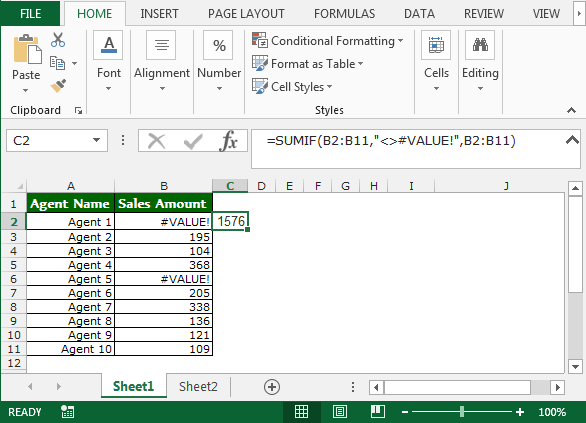There is another formula to return the same result as shown in above image.

• Select the cell C2.
• Write the formula =IF(ISERROR(B2),0,B2)
• Press Enter on your keyboard.
• The function will return the value; thereby, ignoring the errors.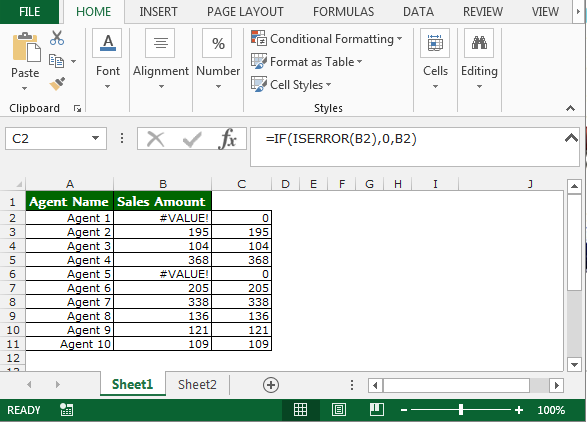• Write the formula in cell D2.
• =SUM(C2:C11), press enter on your keyboard.
• The function will return the total value.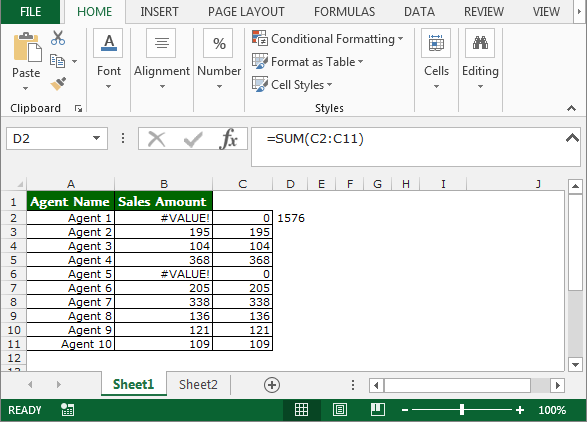Take away:-

• To return the sum by ignoring the error.
• How we can use Excel functions and formulas to solve this problem.1.